# Colleges with the lowest SAT scores in Maryland

Top 10 colleges in Maryland with the lowest SAT scores
Looking for the colleges with the lowest SAT scores in Maryland? Well you're in luck! We've compiled a national college database and have created a list of the top 10 universities with the lowest SAT scores in Maryland below. If you are not a good test taker or worried about your test scores, this list is for you. These are the schools whose applicants had the lowest average SAT scores in Maryland, which means that you can get into these colleges with a lower SAT score. We also include each college's ACT scores and acceptance rate so that you can see where you would have the easiest time getting in. Read on to find out more.

## Bowie State University SAT scores

The average SAT score for Bowie State University is 940.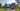The average SAT score of 940 breaks down into:

• SAT math: 460

The average ACT score for Bowie State University is 17 and their acceptance rate is 38%.

## Coppin State University SAT scores

The average SAT score for Coppin State University is 950.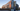The average SAT score of 950 breaks down into:

• SAT math: 470

The average ACT score for Coppin State University is 17 and their acceptance rate is 53.5%.

## University of Baltimore SAT scores

The average SAT score for University of Baltimore is 1040.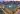The average SAT score of 1040 breaks down into:

• SAT math: 510

The average ACT score for University of Baltimore is 20 and their acceptance rate is 60%.

## Frostburg State University SAT scores

The average SAT score for Frostburg State University is 1060.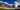The average SAT score of 1060 breaks down into:

• SAT math: 520

The average ACT score for Frostburg State University is 20 and their acceptance rate is 72.3%.

## Mount Saint Mary's University SAT scores

The average SAT score for Mount Saint Mary's University is 1080.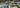The average SAT score of 1080 breaks down into:

• SAT math: 530

The average ACT score for Mount Saint Mary's University is 21 and their acceptance rate is 64.2%.

## Towson University SAT scores

The average SAT score for Towson University is 1140.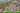The average SAT score of 1140 breaks down into:

• SAT math: 560

The average ACT score for Towson University is 23 and their acceptance rate is 76.3%.

## McDaniel College SAT scores

The average SAT score for McDaniel College is 1154.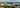The average SAT score of 1154 breaks down into:

• SAT math: 570

The average ACT score for McDaniel College is 24 and their acceptance rate is 85%.

## Hood College SAT scores

The average SAT score for Hood College is 1160.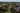The average SAT score of 1160 breaks down into:

• SAT math: 570

The average ACT score for Hood College is 24 and their acceptance rate is 71.4%.

## Saint Mary's College of Maryland SAT scores

The average SAT score for Saint Mary's College of Maryland is 1180.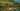The average SAT score of 1180 breaks down into:

• SAT math: 580

The average ACT score for Saint Mary's College of Maryland is 25 and their acceptance rate is 80.1%.

## Washington College SAT scores

The average SAT score for Washington College is 1180.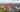The average SAT score of 1180 breaks down into:

• SAT math: 580